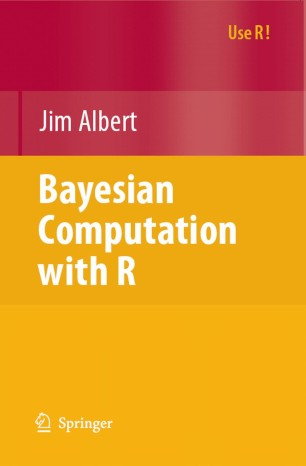Advertisement

© 2007

# Bayesian Computation with R

• Jim AlbertTextbook

Part of the Use R! book series (USE R)

## Table of contents

1. Front Matter
Pages i-x
2. Pages 1-17
3. Pages 19-37
4. Pages 39-56
5. Pages 57-74
6. Pages 75-99
7. Pages 101-135
8. Pages 137-161
9. Pages 163-185
10. Pages 187-210
11. Pages 211-236
12. Pages 237-258
13. Back Matter
Pages 259-267

## About this book

### Introduction

There has been a dramatic growth in the development and application of Bayesian inferential methods. Some of this growth is due to the availability of powerful simulation-based algorithms to summarize posterior distributions. There has been also a growing interest in the use of the system R for statistical analyses. R's open source nature, free availability, and large number of contributor packages have made R the software of choice for many statisticians in education and industry.

Bayesian Computation with R introduces Bayesian modeling by the use of computation using the R language. The early chapters present the basic tenets of Bayesian thinking by use of familiar one and two-parameter inferential problems. Bayesian computational methods such as Laplace's method, rejection sampling, and the SIR algorithm are illustrated in the context of a random effects model. The construction and implementation of Markov Chain Monte Carlo (MCMC) methods is introduced. These simulation-based algorithms are implemented for a variety of Bayesian applications such as normal and binary response regression, hierarchical modeling, order-restricted inference, and robust modeling. Algorithms written in R are used to develop Bayesian tests and assess Bayesian models by use of the posterior predictive distribution. The use of R to interface with WinBUGS, a popular MCMC computing language, is described with several illustrative examples.

This book is a suitable companion book for an introductory course on Bayesian methods and is valuable to the statistical practitioner who wishes to learn more about the R language and Bayesian methodology. The LearnBayes package, written by the author and available from the CRAN website, contains all of the R functions described in the book.

Jim Albert is Professor of Statistics at Bowling Green State University. He is Fellow of the American Statistical Association and is past editor of The American Statistician. His books include Ordinal Data Modeling (with Val Johnson), Workshop Statistics: Discovery with Data, A Bayesian Approach (with Allan Rossman), and Bayesian Computation using Minitab.

### Keywords

Bayesian Inference Hierarchical modeling Markov chain Monte Carlo Monte Carlo Monte Carlo method Regression STATISTICA WinBUGS algorithms linear regression model modeling simulation

### Editors and affiliations

• Jim Albert
• 1
1. 1.Bowling Green State UniversityUSA

### Bibliographic information

• Book Title Bayesian Computation with R
• Authors Jim Albert
• Series Title Use R!
• DOI https://doi.org/10.1007/978-0-387-71385-4
• Copyright Information Springer Science+Business Media, LLC 2007
• Publisher Name Springer, New York, NY
• eBook Packages Mathematics and Statistics Mathematics and Statistics (R0)
• Softcover ISBN 978-0-387-71384-7
• eBook ISBN 978-0-387-71385-4
• Edition Number 1
• Number of Pages X, 268
• Number of Illustrations 0 b/w illustrations, 0 illustrations in colour
• Topics
• Buy this book on publisher's site
Industry Sectors
Pharma
Biotechnology
Finance, Business & Banking
IT & Software
Consumer Packaged Goods
Oil, Gas & Geosciences
Engineering

## Reviews

From the reviews:

The book is a concise presentation of a wide range of Bayesian inferential problems and the computational methods to solve them. The detailed and thorough presentation style, with complete R code for the examples, makes it a welcome companion to a theoretical text on Bayesian inference.... Smart students of statistics will want to have both R and Bayesian inference in their portfolio. Jim Albert's book is a good place to try out R while learning various computational methods for Bayesian inference. (Jouni Kerman, Teh American Statistician, February 2009, Vol. 63, No.1)

"This is a compact text, with 11 chapters. Overall it is well written and contains a plethora of interesting examples … . Each chapter ends with short notes on further reading, a summary of R commands that are introduced, and a collection of excellent exercises to test understanding of the material. … this book would be a useful companion to an introductory Bayesian text in a classroom setting or as a primer on R for a Bayesian practitioner." (John Verzani, SIAM reviews, Vol. 50 (4), December, 2008)

"This textbook is a compact introduction to modern computational Bayesian statistics. Without caring too much about mathematical details, the author gives an overall view of the main problems in statistics … . The examples and the applications provided are intended for a general audience of students." (Mauro Gasparini, Zentralblatt MATH, Vol. 1160, 2009)

“A book about Bayesian computation is highly welcome. … The book contains many interesting examples and is especially stimulating for students who start writing their own Bayesian programs. … This book serves this demand of students perfectly. … Thus, the book can be highly recommended for all introductory Bayes courses, preferably if the students had a statistics course with an introduction to R (or Splus) before.”­­­ (Wolfgang Polasek, Statistical Papers, Vol. 52, 2011)